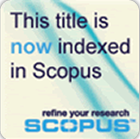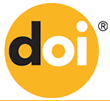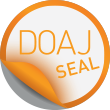﻿ PAKISTAN VETERINARY JOURNAL
PAKISTAN
VETERINARY
JOURNAL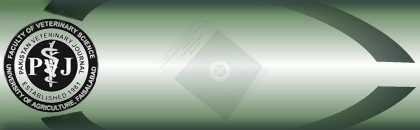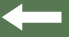Pak Vet J, 2013, 33(1): 23-26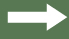Effect of Milk Yield on Economic Profitability of Holstein Friesian Cows under Intensive Production System in Egypt

Animal Production Department, Faculty of agriculture, Kafrelsheikh University, PC: 33516, Kafrelsheikh, Egypt

## Abstract

Data of productive and reproductive traits of 1961 of lactation records that obtained from 763 Dutch Friesian cows grew up at Wadi El-Sharkia farm, El-Salheia, Egypt. These data were analyzed to empirical comparison between the profitability of three herds under intensive production system in Egypt. The second herd (H2) produced average milk yield 8619 kg/lactation, followed by first herd (H1) (5138 kg/lactation) which decreased than the H2 by at least one standard deviation (SD = 2935 kg). The third herd (H3) produced high milk yield equal the average of H2 plus at least one standard deviation. Traits Studied were milk yield per day (M/D, kg), total milk yield (TMY, kg), lactation period (LP, day), dry period (DP, day), annual milk yield (AMY, kg), days open (DO, day), number of services per conception (NSPC), calving interval (CI, day), number of lactation completed (NLC) and age at first calving (AFC). Least squares analysis of variance showed highly significant (P<0.01) effects of all factors on all traits studied except the effect of month and year of calving on NSPC that have non-significant. For comparing between the three herds, the deterministic model was used to estimate the annual gross margin and benefit/cost ratio as economic parameters. Price of inputs and outputs were based on market and farm gate prices during the period from 1998 to 2007. DO, CI and NSPC were increased in H3 vs. H2 and H1, indicating poorer reproductive efficiency of high yielding herd. Moreover, H3 gave 1.03 and 1.5 parity less for each cow than that of H2 and H1, respectively. Economic evaluations indicated that the annual variable cost were (L.E) (Egyptian pound = 0.17 USD and = 0.13 EUR) 5136, 6910 and 7845 of H1, H2 and H3, respectively. However, the annual gross margin of H3 was higher than that of H1 and H2 by 79 and 24%, respectively, and the benefit/cost ratio of H3 are LE 1.90 relative to 1.63 and 1.68 for H1 and H2, respectively. The profit per cow during the lifetime production of H3 was 72 and 19.04% more than of H1 and H2, respectively. It concluded that under intensive production system, extension of calving interval for high yielding herds seem more profitable than the herds that have shorter calving interval and lactation period.

Key words: Benefit/cost ratio, Deterministic, Gross margin, Intensive, Profitable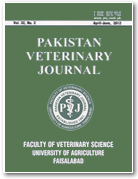### ISSN 0253-8318 (Print)ISSN 2074-7764 (Online)